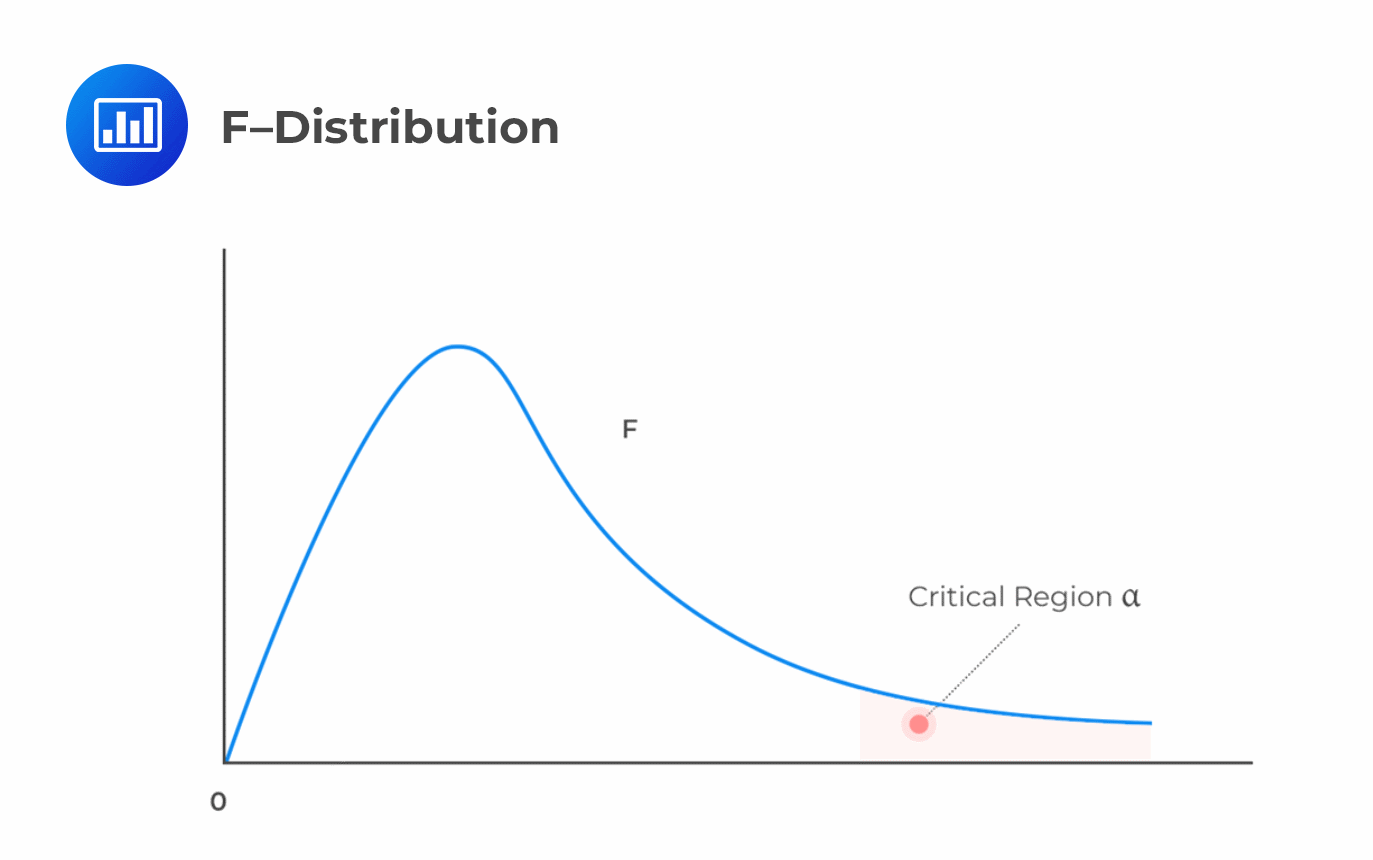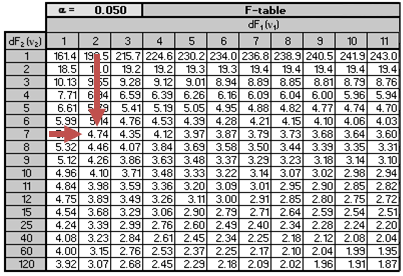# The F-statistic

The F-test determines whether all the independent variables help explain the dependent variable. It is a test of regression’s overall significance that involves testing the null hypothesis that all the slope coefficients in the regression are jointly equal to zero against the alternative hypothesis that at least one slope coefficient is not equal to 0:

$$H_{0}: b_{1}=b_{2}=…=b_{k}=0$$ versus $$H_{a}:$$ at least one $$b_{j}≠0$$

It is imperative to understand that we cannot use the t-test to determine whether every slope coefficient is zero. This is because individual tests do not account for interactions among the dependent variables.

The following inputs are required to determine the test statistic for the null hypothesis.

i. The total number of observations, $$n$$.

ii. The total number of slope coefficients to be estimated, $$k+1$$, where $$k$$ = number of slope coefficients.

iii. The Residual Sum of Squares (SSE), which is the unexplained variation.

iv. The Regression Sum of Squares (RSS), which is the explained variation.

The F-statistic (which is a one-tailed test) is computed as:

\begin{align*}F&=\frac{\bigg(\frac{RSS}{k}\bigg)}{\bigg(\frac{SSE}{n-(k+1)}\bigg)}\\&=\frac{\text{Mean Regression Sum of Squares (MSR)}}{\text{Mean Squared Error (MSE)}}\end{align*}

Where:

• RSS = Regression Sum of Squares
• SSE = Sum of Squared Errors
• n = number of observations
• k = number of independent variables

A large value of F implies that the regression model does a good job in explaining variation in the dependent variable. On the other hand, the value of F will be zero when the independent variables do not explain the dependent variable at all.

The F-test is denoted as $$F_{k, (n-(k+1))}$$. The test should have $$k$$ degrees of freedom in the numerator and $$n-(k+1)\_ degrees of freedom in the denominator. ## Decision Rule We reject the null hypothesis at a given significance level, \(α$$, if the calculated value of $$F$$ is greater than the upper critical value of the one-tailed $$F$$ distribution with the specified degrees of freedom.Reject $$H_0$$ if F-statistic $$>F_{c}$$ (critical value)

### Example: Calculating and Interpreting the F-statistic

The following ANOVA table presents the output of the multiple regression analysis of the price of the US Dollar index on the inflation rate and real rate of interest.

$$\textbf{Exhibit 1: ANOVA Table}$$

$$\small{\begin{array}{c|c|c|c|c|c}\hline{}& \textbf{df} & \textbf{SS} & \textbf{MS} & \textbf{F} & \textbf{Significance F}\\ \hline\text{Regression} & 2 & 432.2520 & 216.1260 & 7.5405 & 0.0179\\ \hline\text{Residual} & 7 & 200.6349 & 28.6621 & {}& {}\\ \hline\text{Total} & 9 & 632.8869 & & &{}\\ \hline\end{array}}$$

Test the null hypothesis that all of the independent variables are equal to zero at the 5% significance level.

#### Solution

We test the null hypothesis:

$$H_{0}: \beta_{1}=\beta_{2}=0$$ versus $$H_a$$: at least one $$b_j≠0$$.

Number of slope coefficients $$(k)=2$$.

Degrees of freedom in the denominator = $$n-(k+1)=10-(2+1)=7$$.

SSE = 200. 6349

Thus,

\begin{align*}F&=\frac{\bigg(\frac{RSS}{k}\bigg)}{\bigg(\frac{SSE}{n-(k+1)}\bigg)}\\&=\frac{\frac{432.2520}{2}}{\frac{200.6349}{7}}\\&=7.5405\end{align*}

For  $$\alpha=0.05$$, the critical value of F with $$k= 2$$ and $$(n – k – 1) = 7$$ degrees of freedom $$F_{0.05,2,7}$$ is approximately 4.74.The calculated value of the F-test statistic, 7.5405, is greater than the critical value of F, 4. 737. We, therefore, reject the null hypothesis that coefficients of both independent variables equal 0.

Additionally, notice that from the ANOVA table, the column “Significance F” reports a p-value of 0.0179, which is less than 0.05. The p-value implies that the smallest level of significance at which the null hypothesis can be rejected is 0.0179.

## Question

Adil Suleman, CFA, wishes to establish the possible drivers of a company’s percentage return on capital (ROC). Suleman identifies performance measures such as the profit margin (%), sales, and debt ratio as possible drivers of ROC.

He then regresses the ROC against the three independent variables: Sales, profit margin, and debt ratio. The output from a statistical software package is presented below:

• Observations: 25.
• The total sum of squares: 80.16.
• Sum of squared errors: 30.0545.

Suleman proceeds to test the null hypothesis at the 5% significance level that all three of the independent variables are equal to zero.

The F-statistic for testing whether the slope coefficient is equal to zero is closest to:

A11.67.

B. 18.67.

C. 19.56.

### Solution

\begin{align*}F&=\frac{\text{(Mean Regression Sum of Squares)}}{\text{Mean squared error}}\\&=\frac{(\frac{RSS}{k})}{(\frac{SSE}{n-(k+1)})}\end{align*}

$$\text{RSS}=\text{SST}-\text{SSE}$$

\begin{align*}\text{RSS}&=80.16-30.0545\\&=50.1055\end{align*}

\begin{align*}\text{Mean Regression Sum of Squares}&=\frac{50.1055}{3}\\&=16.7018\end{align*}

\begin{align*}\text{Mean Squared Error}&= \frac{30.0545}{25-3-1}\\&=1.4312\end{align*}

\begin{align*}F&=\frac{16.7018}{1.4312}\\&=11.67\end{align*}

LOS 2 (g) Calculate and interpret the Fstatistic, and describe how it is used in regression analysis.

Shop CFA® Exam Prep

Offered by AnalystPrepLevel I
Level II
Level III
All Three Levels
Featured Shop FRM® Exam PrepFRM Part I
FRM Part II
FRM Part I & Part II
Learn with Us

Subscribe to our newsletter and keep up with the latest and greatest tips for success
Shop Actuarial Exams PrepExam P (Probability)
Exam FM (Financial Mathematics)
Exams P & FMGMAT® Complete CourseDaniel Glyn
2021-03-24
I have finished my FRM1 thanks to AnalystPrep. And now using AnalystPrep for my FRM2 preparation. Professor Forjan is brilliant. He gives such good explanations and analogies. And more than anything makes learning fun. A big thank you to Analystprep and Professor Forjan. 5 stars all the way!michael walshe
2021-03-18
Professor James' videos are excellent for understanding the underlying theories behind financial engineering / financial analysis. The AnalystPrep videos were better than any of the others that I searched through on YouTube for providing a clear explanation of some concepts, such as Portfolio theory, CAPM, and Arbitrage Pricing theory. Watching these cleared up many of the unclarities I had in my head. Highly recommended.Nyka Smith
2021-02-18
Every concept is very well explained by Nilay Arun. kudos to you man!2021-02-13